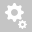##Recently viewed tickets# Midas Civil moving analysis reactions from trains with Railway dynamic factor

Question:

Railway dynamic factor can be specifically defined for any element in the model. But reactions forces are calculated in nodes. So it is not clear what dynamic factor was chosen for node which is common for two elements with different dynamic factors.

Simple example if we have longitudinal element and transverse beam element with one common nodal support. Acc. to code there should be different dynamic factor. What factor is used in calculation? Can you describe in detail how program picks the dynamic factor?

objevil jsem problém v programu MIDAS Civil při výpočtu reakcí od pohyblivého zatížení drážním vozidlem dle EC.

Also user would like to know in detail how program deals with dynamic factors. In help there is very little info about that.

Hello,

Let me explain you with the help of example.
A steel composite bridge -grillage was made in which moving load as per Eurocode was applied. Standard Load model 71  railway vehicle was mage to run on the centre of the bridge.Bridge was symmetric. Also, determinant length of the bridge was kept as 12 m (just assumption) and standard maintenance was chosen. Now, for this, dynamic factor value is 1.3917.
Now, the result for reaction at node 681 is 1147.5kN for non-dynamic case vs 1597kN (=1147.5 x 1.3917) for dynamic case as shown below.Further, when elemental dynamic factor are changed, the reaction is calculated as per system dynamic factor.

Now, in next case, the dynamic factor for 2 adjacent elements ( 323 & 324) were made different and determinant length was kept as 4m (just an assumption) for element 303. So dynamic factor for element 323 calculated was 1.93 and element 324 will remain the same as 1.3917.The results for j end for element 323 and i-end of element 324 for non-dynamic load factor case is 3217Knm.

So, j end of the element 323 will produce result as per 1.93 dynamic factor and i-end of element 324 will produce the results as per 1.3917 as shown below.For j end of element 323, My is 6209 (=1.93x 3216) and i-end of the element 324, My is 4471 (=1.39 x 3216).
Hence, dynamic factor for element modifies the results for the elements at both the end and node having 2 elements with different dynamic factor shows different result. Nodes results on itself has no significance. Actually, it displays the results for the elements.

Quesiton: how program deals with dynamic factors. In help there is very little info about that.
Answer: Results for all the moving load are firstly calculated for normal vehicular load and then, dynamic factor are applied to the calculated result and then displayed to us. As explained earlier, the elemental force for 323 and 324 is calculated for moving load and then, the dynamic factor and all other factors are applied as per the definition of each and every element.

The model file has been attached for further review.
without dynamic factor is model where moving load is applied without dynamic factor.
dynamic factor is model where moving load is applied with dynamic load.\
Creation date: 6/8/2021 1:42 PM (jatin2010)      Updated: 6/8/2021 1:42 PM ()